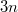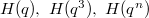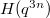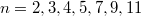﻿

### Jacobi-Sohncke Type Mixed Modular Equations And Their Applications

#### Abstract

In this paper, we establish Jacobi-Sohncke type several new mixed modular equations for composite degrees 1, 3,$n$ and$3n$. As an application, we establish the modular relations between the Ramanujan-Selberg continued fractions$H(q),\ H(q^3),\ H(q^n)$ and$H(q^{3n})$ for$n=2,3,4,5,7,9,11$ and also we obtain congruence relations for color overpartitions of$n$ with odd parts.

DOI Code: 10.1285/i15900932v36n1p37

Keywords: Modular equation; Theta-function; continued fraction

Full Text: PDF